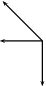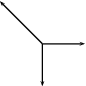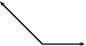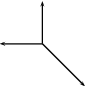# 10.3 Components  (Page 3/3)

 Page 3 / 3

## Adding and subtracting components of vectors

1. Harold walks to school by walking 600 m Northeast and then 500 m N 40 o W. Determine his resultant displacement by means of addition of components of vectors.
2. A dove flies from her nest, looking for food for her chick. She flies at a velocity of 2 m $·$ s ${}^{-1}$ on a bearing of 135 ${}^{o}$ in a wind with a velocity of 1,2 m $·$ s ${}^{-1}$ on a bearing of 230 ${}^{o}$ . Calculate her resultant velocity by adding the horizontal and vertical components of vectors.

## Vector multiplication

Vectors are special, they are more than just numbers. This means that multiplying vectors is not necessarily the same as just multiplying their magnitudes. There are two different types of multiplication defined for vectors. You can find the dot product of two vectors or the cross product.

The dot product is most similar to regular multiplication between scalars. To take the dot product of two vectors, you just multiply their magnitudes to get out a scalar answer. The mathematical definition of the dot product is:

$\stackrel{\to }{a}•\stackrel{\to }{b}=|\stackrel{\to }{a}|·|\stackrel{\to }{b}|cos\theta$

Take two vectors $\stackrel{\to }{a}$ and $\stackrel{\to }{b}$ :

You can draw in the component of $\stackrel{\to }{b}$ that is parallel to $\stackrel{\to }{a}$ :

In this way we can arrive at the definition of the dot product. You find how much of $\stackrel{\to }{b}$ is lined up with $\stackrel{\to }{a}$ by finding the component of $\stackrel{\to }{b}$ parallel to $\stackrel{\to }{a}$ . Then multiply the magnitude of that component, $|\stackrel{\to }{b}|$ $cos\theta$ , with the magnitude of $\stackrel{\to }{a}$ to get a scalar.

The second type of multiplication, the cross product, is more subtle and uses the directions of the vectors in a more complicated way. The cross product of two vectors, $\stackrel{\to }{a}$ and $\stackrel{\to }{b}$ , is written $\stackrel{\to }{a}×\stackrel{\to }{b}$ and the result of this operation on $\stackrel{\to }{a}$ and $\stackrel{\to }{b}$ is another vector. The magnitude of the cross product of these two vectors is:

$|\stackrel{\to }{a}×\stackrel{\to }{b}|=|\stackrel{\to }{a}||\stackrel{\to }{b}|sin\theta$

We still need to find the direction of $\stackrel{\to }{a}×\stackrel{\to }{b}$ . We do this by applying the right hand rule .

Method: Right Hand Rule

2. Point your index finger in the direction of $\stackrel{\to }{a}$ .
3. Point the middle finger in the direction of $\stackrel{\to }{b}$ .
4. Your thumb will show the direction of $\stackrel{\to }{a}×\stackrel{\to }{b}$ .

## Summary

1. A scalar is a physical quantity with magnitude only.
2. A vector is a physical quantity with magnitude and direction.
3. Vectors may be represented as arrows where the length of the arrow indicates the magnitude and the arrowhead indicates the direction of the vector.
4. The direction of a vector can be indicated by referring to another vector or a fixed point (eg. 30 ${}^{\circ }$ from the river bank); using a compass (eg. N 30 ${}^{\circ }$ W); or bearing (eg. 053 ${}^{\circ }$ ).
5. Vectors can be added using the head-to-tail method, the parallelogram method or the component method.
6. The resultant of a number of vectors is the single vector whose effect is the same as the individual vectors acting together.

## End of chapter exercises: vectors

1. An object is suspended by means of a light string. The sketch shows a horizontal force $F$ which pulls the object from the vertical position until it reaches an equilibrium position as shown. Which one of the following vector diagrams best represents all the forces acting on the object?
 A B C D2. A load of weight $W$ is suspended from two strings. ${F}_{1}$ and ${F}_{2}$ are the forces exerted by the strings on the load in the directions show in the figure above. Which one of the following equations is valid for this situation?
1. $\phantom{\rule{0.277778em}{0ex}}\phantom{\rule{0.277778em}{0ex}}$ $W={F}_{1}^{2}+{F}_{2}^{2}$
2. $\phantom{\rule{0.277778em}{0ex}}\phantom{\rule{0.277778em}{0ex}}$ ${F}_{1}sin{50}^{\circ }={F}_{2}sin{30}^{\circ }$
3. $\phantom{\rule{0.277778em}{0ex}}\phantom{\rule{0.277778em}{0ex}}$ ${F}_{1}cos{50}^{\circ }={F}_{2}cos{30}^{\circ }$
4. $\phantom{\rule{0.277778em}{0ex}}\phantom{\rule{0.277778em}{0ex}}$ $W={F}_{1}+{F}_{2}$
3. Two spring balances $P$ and $Q$ are connected by means of a piece of string to a wall as shown. A horizontal force of 100 N is exerted on spring balance Q. What will be the readings on spring balances $P$ and $Q$ ?
 P Q A 100 N 0 N B 25 N 75 N C 50 N 50 N D 100 N 100 N
4. A point is acted on by two forces in equilibrium. The forces
1. have equal magnitudes and directions.
2. have equal magnitudes but opposite directions.
3. act perpendicular to each other.
4. act in the same direction.
5. A point in equilibrium is acted on by three forces. Force ${F}_{1}$ has components 15 N due south and 13 N due west. What are the components of force ${F}_{2}$ ?
1. 13 N due north and 20 due west
2. 13 N due north and 13 N due west
3. 15 N due north and 7 N due west
4. 15 N due north and 13 N due east
6. Which of the following contains two vectors and a scalar?
1. distance, acceleration, speed
2. displacement, velocity, acceleration
3. distance, mass, speed
4. displacement, speed, velocity
7. Two vectors act on the same point. What should the angle between them be so that a maximum resultant is obtained?
1. 0 ${}^{\circ }$
2. 90 ${}^{\circ }$
3. 180 ${}^{\circ }$
4. cannot tell
8. Two forces, 4 N and 11 N, act on a point. Which one of the following cannot be the magnitude of a resultant?
1. 4 N
2. 7 N
3. 11 N
4. 15 N

## End of chapter exercises: vectors - long questions

1. A helicopter flies due east with an air speed of 150 km.h ${}^{-1}$ . It flies through an air current which moves at 200 km.h ${}^{-1}$ north. Given this information, answer the following questions:
1. In which direction does the helicopter fly?
2. What is the ground speed of the helicopter?
3. Calculate the ground distance covered in 40 minutes by the helicopter.
2. A plane must fly 70 km due north. A cross wind is blowing to the west at 30 km.h ${}^{-1}$ . In which direction must the pilot steer if the plane flies at a speed of 200 km.h ${}^{-1}$ in windless conditions?
3. A stream that is 280 m wide flows along its banks with a velocity of 1.80m.s ${}^{-1}$ . A raft can travel at a speed of 2.50 m.s ${}^{-1}$ across the stream. Answer the following questions:
1. What is the shortest time in which the raft can cross the stream?
2. How far does the raft drift downstream in that time?
3. In what direction must the raft be steered against the current so that it crosses the stream perpendicular to its banks?
4. How long does it take to cross the stream in part c?
4. A helicopter is flying from place $X$ to place $Y$ . $Y$ is 1000 km away in a direction ${50}^{\circ }$ east of north and the pilot wishes to reach it in two hours. There is a wind of speed 150 km.h ${}^{-1}$ blowing from the northwest. Find, by accurate construction and measurement (with a scale of $1\phantom{\rule{3.33333pt}{0ex}}\mathrm{cm}=50\phantom{\rule{3.33333pt}{0ex}}{\mathrm{km}.\mathrm{h}}^{-1}$ ), the
1. the direction in which the helicopter must fly, and
2. the magnitude of the velocity required for it to reach its destination on time.
5. An aeroplane is flying towards a destination 300 km due south from its present position. There is a wind blowing from the north east at 120 km.h ${}^{-1}$ . The aeroplane needs to reach its destination in 30 minutes. Find, by accurate construction and measurement (with a scale of $1\phantom{\rule{3.33333pt}{0ex}}\mathrm{cm}=30\phantom{\rule{3.33333pt}{0ex}}{\mathrm{km}.\mathrm{s}}^{-1}$ ), or otherwise,
1. the direction in which the aeroplane must fly and
2. the speed which the aeroplane must maintain in order to reach the destination on time.
6. An object of weight $W$ is supported by two cables attached to the ceiling and wall as shown. The tensions in the two cables are ${T}_{1}$ and ${T}_{2}$ respectively. Tension ${T}_{1}=1200$  N. Determine the tension ${T}_{2}$ and weight $W$ of the object by accurate construction and measurement or by calculation.
7. In a map-work exercise, hikers are required to walk from a tree marked A on the map to another tree marked B which lies 2,0 km due East of A. The hikers then walk in a straight line to a waterfall in position C which has components measured from B of 1,0 km E and 4,0 km N.
1. Distinguish between quantities that are described as being vector and scalar .
2. Draw a labelled displacement-vector diagram (not necessarily to scale) of the hikers' complete journey.
3. What is the total distance walked by the hikers from their starting point at A to the waterfall C?
4. What are the magnitude and bearing, to the nearest degree, of the displacement of the hikers from their starting point to the waterfall?
8. An object $X$ is supported by two strings, $A$ and $B$ , attached to the ceiling as shown in the sketch. Each of these strings can withstand a maximum force of 700 N. The weight of $X$ is increased gradually.
1. Draw a rough sketch of the triangle of forces, and use it to explain which string will break first.
2. Determine the maximum weight of $X$ which can be supported.
9. A rope is tied at two points which are 70 cm apart from each other, on the same horizontal line. The total length of rope is 1 m, and the maximum tension it can withstand in any part is 1000 N. Find the largest mass ( $m$ ), in kg, that can be carried at the midpoint of the rope, without breaking the rope. Include a vector diagram in your answer.

What is a vector
vector is anything that has both a direction and a magnitude .they are usually drawn as pointed arrows ,the length of which represents a vector's magnitude
Tetteh
what is electronics?
Edward
how to calculate the reading on voltmeter or ammeter
why is HCl considered a strong acid
it dissociate almost completely
Tetteh
what is metal displacement
what is an electric field?
is the charge of an electron always 1,6 ×10^-19? and the mass is always 9,1×10^-13?
how to calculate a distance between charges
juss apply the formula of the Electrostatic force
Noluthando
please what's the simplest way to derived Schrodinger's wavelength equation
Maimo
how to show polarity
what can ii do to pass physics
Use previous years question papers to understand how questions are answered and asked
Joseph
but it hard ii am a slow learner
Slindile
Then the best thing to do is that immediately you are done reading through a certain topic, and you think you understood everything in that topic that's when you can use previous question papers and answer questions related to the topic. I think that's not difficult
Joseph
mm ii will try
Slindile
Good
Joseph
Here's a tip in reading textbooks, don't read it like a novel. First, flip through the pages—scan the chapter that you wanted to read. Second, go to the end of the chapter. Usually, there's a quiz at the end, so if will give you the important information that you need to know.
Sarah
Third, go to the beginning of the chapter and read through the words that were printed in bold: Titles, subtitles, headings, important words —because it helps to break down information.
Sarah
Fourth, read the first sentence of the chapter—if it is written by a good author; therefore, it will also have a good introduction. Check also the last sentence of the chapter to sum it up. Finally, read the whole chapter. You won't read it twice anymore.
Sarah
It looks hard, cause there are so much to do but read it thoroughly, it's easy and it will help you to save time and comprehend better. If you don't really have interest on reading—there are various of videos in youtube😊
Sarah
Oh, so em dashes turn into question marks :' (( nvm. Goodluck to all of us!
Sarah
How many ways can we calculate the empirical formula
How is current divided between resistors in parallel
Joseph
1/R=1/R+1/R
Slindile
tanx
Joseph
ii know 2 ways
Slindile
what's the other way
Joseph
What is the meaning of Coulomb's law
Electronic magnetic field
what is an emf ?
electromotive force
Tetteh
what are moments of a force
the moment of a force" is a measure of a tendency to cause a body to rotate about a specific point or axis....a moment is due to force not having equal and opposite force directly along its line of action.
Bhuboy
how to calculate the magnitude of the force of repulsionBy OpenStaxBy OpenStaxBy Madison ChristianBy Sam LuongBy Jonathan LongBy OpenStaxBy OpenStaxBy Vanessa SoledadBy Angela EckmanBy OpenStax